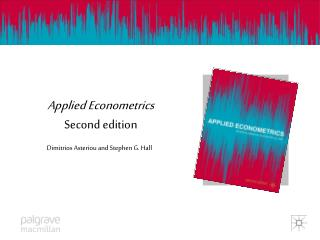DownloadDownload PresentationApplied Econometrics Second edition

# Applied Econometrics Second edition

Download Presentation## Applied Econometrics Second edition

- - - - - - - - - - - - - - - - - - - - - - - - - - - E N D - - - - - - - - - - - - - - - - - - - - - - - - - - -
##### Presentation Transcript

1. Applied EconometricsSecond edition Dimitrios Asteriou and Stephen G. Hall

2. MULTIPLE REGRESSION 1. The Multiple Regression Model 2. The OLS Method of Estimation 3. The R2 and the Adjusted R2 4. Hypothesis Testing 5. How to Estimate a Simple Regression in EViews

3. Learning Objectives • Derive mathematically the regression coefficients of a multiple regression model. • Understand the difference between the R2 and the adjusted R2 for a multiple regression model. • Appreciate the importance of the various selection criteria for the best regression model. • Conduct hypothesis testing and test linear restrictions, omitted and redundant variables as well as the overall significance of the explanatory variables.

4. Learning Objectives (2) • Obtain the output of a multiple regression estimation using econometric software. • Interpret and discuss the results of a multiple regression estimation output.

5. Multiple Regression Derivation of the OLS • The three variables case (explain on board) • The k-variables case (explain on board) • Requires matrix algebra and it is quite complicated • Luckily Eviews, Mfit and Stata give results very quickly and efficiently (always correct calculations)

6. R2and adjusted R2 • R2measures goodness of fit as in Simple Regression • However, it cannot be used for comparing two different equations containing different • numbers of explanatory variables. • When adding more explanatory variables R2, will always be increased. • Therefore we need a different measure (the adjusted R2)

7. R2and adjusted R2 • R2 = ESS/TSS = 1 − RSS/TSS • Adj R2= • Similar to R2but adjusts for degrees of freedom

8. Criteria for Model Selection • Akaike Information Criterion (AIC) • Finite Prediction Error (FPE) • Schwarz Bayesian Criterion (SBC) • Hannan and Quin Criterion (HQC)

9. Multiple Regression in EViews • Step 1 Open EViews. • Step 2 Click File/New/Workfilein order to create a new file or File/Open to open an existing file. • Step 3 Enter the data • Step 4 Type in the EViews command line: ls y c x2 x3 . . . xk (press ‘enter’)

10. Hypothesis Testing • Testing Individual Coefficients (t-tests) • Testing for Linear Restrictions (Wald Test) • Cobb Douglas Production Function • Testing for the Overall Significance (F-test) • Testing for Omitted Variables (Wald Test) • Testing for Redundant Variables (Wald Test) • Explain all the tests on board…